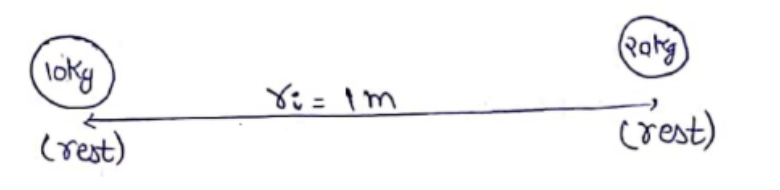Deepak Scored 45->99%ile with Bounce Back Crack Course. You can do it too!

# Solve the following :

Question:

Two small bodies of masses $10 \mathrm{~kg}$ and $20 \mathrm{~kg}$ are kept a distance $1.0 \mathrm{~m}$ apart and released, Assuming that only mutual gravitational forces are acting; find the speeds of the particles when the separation decreases to $0.5 \mathrm{~m}$.

Solution:

$m_{1}=10 \mathrm{~kg}, \quad m_{2}=20 \mathrm{~kg}$

Initially,Finally,

$\underset{\gamma_{f}=0.5 \mathrm{~m}}{\stackrel{10 \mathrm{~kg}}{\rightarrow} \mathrm{O}_{1} \quad V_{2} \text { - 20kf }}$

If both bodies are taken as system.

$\vec{F}_{\text {ext }}=0$

$\vec{P}_{i}=\vec{P}_{f}$

$0+0=10 V_{1}-20 V_{2}$

$10 V_{1}=20 V_{2}$

$V_{1}=2 V_{2}$

Now, applying law of conservation of energy,

$T E_{i}=T E_{f}$

$\frac{-G m_{1} m_{2}}{r_{i}}+0+0=\frac{-G m_{1} m_{2}}{r_{f}}+\frac{1}{2} m_{1} v_{1}^{2}+\frac{1}{2} m_{2} v_{2}^{2}$

$\frac{-6.67 \times 10^{-11} \times 10 \times 20}{1}$

On solving,

$V_{1}=4.2 \times 10^{-5} \mathrm{~m} / \mathrm{s}$

and

$V_{2}=\frac{V_{1}}{2}=2.1 \times 10^{-5} \mathrm{~m} / \mathrm{s}$